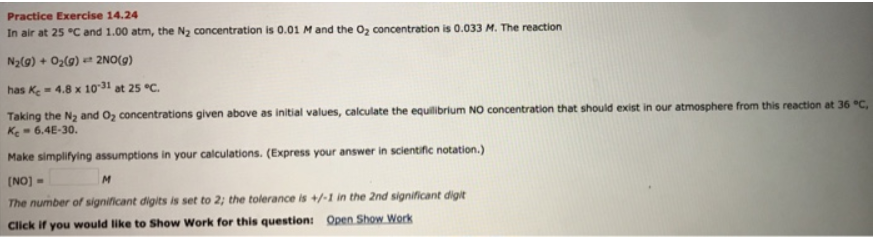# Problem: In air at 25°C and 1.00 atm, the N2 concentration is 0.01 M and the O2 concentration is 0.033 M. The reactionN2(g) + O2(g) ⇌ 2N0(g) has Kc = 4.8 x 10-31 at 25°C.Taking the N2 and O2 concentrations given above as initial values, calculate the equilibrium NO concentration that should exist in our atmosphere from this reaction at 36°C, Kc = 6.4E-30. Make simplifying assumptions in your calculations. (Express your answer in scientific notation.)

###### FREE Expert Solution
90% (285 ratings)###### Problem Details

In air at 25°C and 1.00 atm, the N2 concentration is 0.01 M and the O2 concentration is 0.033 M. The reaction

N2(g) + O2(g) ⇌ 2N0(g)

has Kc = 4.8 x 10-31 at 25°C.

Taking the N2 and O2 concentrations given above as initial values, calculate the equilibrium NO concentration that should exist in our atmosphere from this reaction at 36°C, Kc = 6.4E-30.